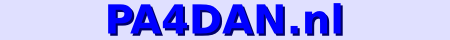Home

Quick info
- Band :
23cm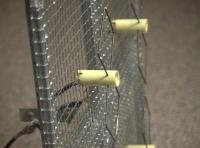• Why?

• A while ago I tried to receive ATV-signals with a single double-quad antenna. The antenna performed very good compared to a simple groundplane antenna.
But signals were still too weak to really make a picture out of it, so I started experimenting with stacking more than one double-quad to get a higher gain.
This is the antenna I came up with :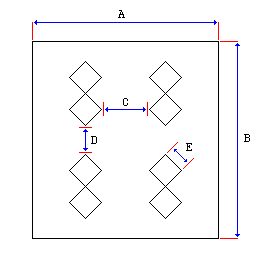Mark Length (in cm) A 35.0 B 42.0 C 11.7 D 2.3 E 5.8

• One single double-quad looks like this :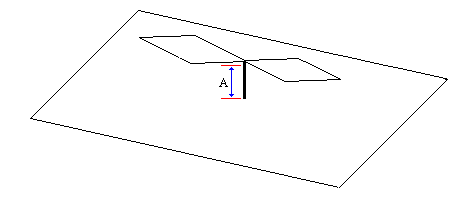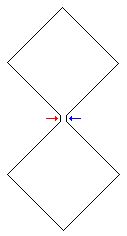The distance between the double-quad and the reflector (shown as 'A' in the picture above), is 3.2 cm.
The red and blue arrows show the place where the inner- and outer-conductor of the coax cable should be connected to the double-quad.

• Phasing the antennas

• The impedance of a single double-quad should be about 50 Ohm. I first connected the 4 antennas parallel using pieces of 50 Ohm cable.
Although the antenna performed better than a single double-quad, the impedances aren't matched, so the VSWR can't possible be very good.
This results in reflections on the cable and thus a lower gain.
So to get maximum gain out of the antenna, I build a phasing-network.

Cable impedances can be transformed with a 1/4 Lambda piece of coax cable, according to the following formula (found on this page [ www.vhfman.freeuk.com ] ) :
Z0 = square root (Z1 * Z2)

Where :
 Z0 is the impedance of the quarter-wave coax cable Z1 is the impedance of the tranceiver Z2 is the impedance of the antenna

Connecting two double-quads in parallel would give an impedance of about 25 Ohm. Knowing this you can calculate that the piece of coax should have an impedance of 35,36 Ohm.
A way to get near to this is to connect two identicle pieces of 75 Ohm TV-coax in parallel. This gives a cable-impedance of 70 : 2 = 35 Ohm.
My phasing network looks like this :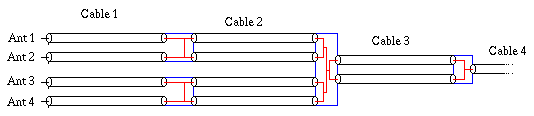Piece Length Cable impedance Cable 1 Any length * 50 Ohm Cable 2 1/4 Lambda 75 Ohm Cable 3 1/4 Lambda 75 Ohm Cable 4 Any length ** 50 Ohm

 * : Any length, but to keep the signals from the different antennas in phase, all 4 pieces of 'Cable 1' must be exactly the same length ** : Any length of coax, connecting the antenna to the tranceiver

Starting at the tranceiver-end, the signal is first transformed by 'Cable 3' and split to the upper and lower pair of double-quads. The lefthand and righthand double-quads are stacked by two transformer of 'Cable 2'.

• Calculating the length of the transformers

• The length of the transformers can be calculated with this formula :
 Lambda = 300 / Frequency Length = Lambda / 4
(With the frequency in MHz and the length in meters)

I calculated the length for 1270 MHz, which gives 5.9cm.
The electricle length of the transformer cable should be 5.9cm. To get the REAL length of the cable, you have to multiply this by the velocity factor of the particular cable you use.
I used TV-coax, but the cable had no markings on it, so I didn't know the velocity factor of it. If you know the type of cable you want to use, you can simply find the velocity factor on the internet.

• Determining the velocity factor of a coax-cable

• To determine the velocity-factor of the cable, I used a spectrum analyser with a tracking-generator.
Take a couple of meters of the cable and strip one end. Leave the other end untouched ( open ).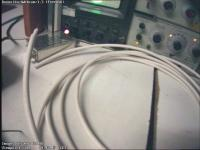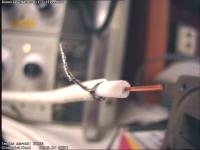Connect output of the tracking generator via a BNC T-adaptor to the input of the spectrum analyser. After you turn on the tracking-generator, you should see a flat line on the analyser.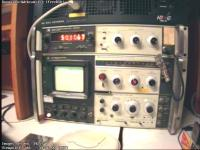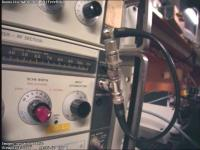Now connect the stripped end of the coax cable to the other input of the BNC-T.
You should now see dips in the spectrum.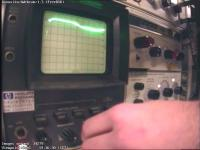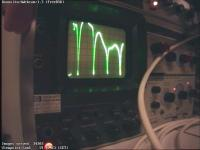Tune the analyser to the first dip and measure it's frequency precisely.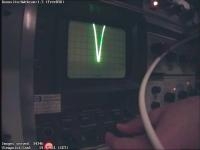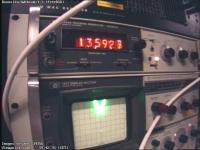At the point of the first dip the electric length of the cable is 1/4 Lambda. At this point, a signal going in to the cable will travel to the end in 1/4 wave-period. At the end the signal bounces at the open end, and starts travelling back into the cable. Again a quarter of a period later, the signal will be at the beginning of the cable again.
By then, the signal has been travelling for exactly half a wave period. At that moment, the signal coming out of the generator is the exact inverse of the signal coming out of the cable. The reflected signal and the generated signal add up to 0. This is the dip you see in the spectrum.

In my measurements I used a piece of cable of 464 cm. The first dip occured at 13.5926 MHz.
1/4 Lambda at 13.5926 MHz is :
300 / 13.5926 * 0.25 = 5.518 m.

The velocity factor of this cable is :
Real length / Electric length = 464 / 551.8 = 0.84

• Some pictures of the finished antenna (without phasing network)

• (click on the images to enlarge them.)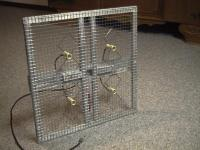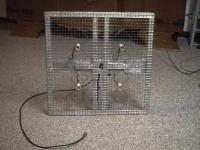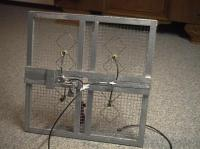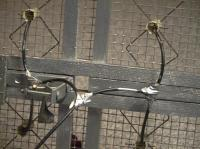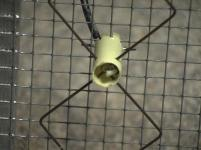Back...

Email me with questions/comments : Daan <Daan @ pa4dan . nl>